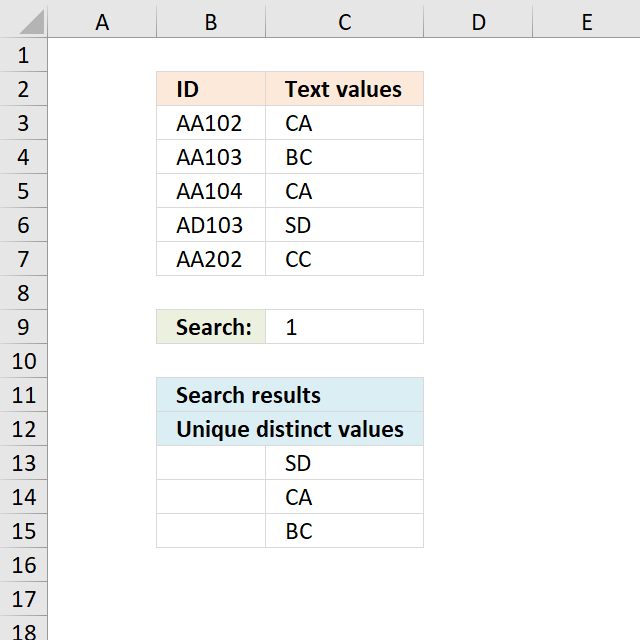Author: Oscar Cronquist Article last updated on December 10, 2018Question: How do I create a unique distinct list where adjacent cell values contain a search string?

AA102 CA
AA103 BC
AA104 CA
AA201 CC

Search string: 1

Unique distinct list
CA
BC
SD

The formula in cellC13 extracts unique distinct values from cell range C3:C7 if adjacent value in column B contains 1.

Formula in C13:

=LOOKUP(2, 1/((COUNTIF(\$C\$12:C12, \$C\$3:\$C\$7)=0)*SEARCH(\$C\$9, \$B\$3:\$B\$7)), \$C\$3:\$C\$7)

copied down as far as needed.

### Explaining formula in cell C13

#### Step 1 - Identify adjacent values containing the search string

The SEARCH function returns a number representing the position of a given string in a cell.

SEARCH(\$C\$9, \$B\$3:\$B\$7)

becomes

SEARCH(1, \$B\$3:\$B\$7)

becomes

SEARCH(1, {"AA102"; "AA103"; "AA104"; "AD103"; "AA202"})

and returns

{3; 3; 3; 3; #VALUE!}

#### Step 2 - Prevent duplicates

The COUNTIF function counts values based on a condition or criteria, if number is 0 (zero) then value has not yet been displayed. The first argument contains an expanding cell reference, when you copy the cell and paste to cells below the cell reference grows. This will make the formula aware of displayed values above current cell.

COUNTIF(\$C\$12:C12, \$C\$3:\$C\$7)=0

becomes

COUNTIF(0,{"CA";"BC";"CA";"SD";"CC"})=0

becomes

{0;0;0;0;0}=0

and returns

{TRUE; TRUE; TRUE; TRUE; TRUE}.

#### Step 3 - Multiply arrays

Use AND logic because both arrays must be TRUE in order to be extracted.

((COUNTIF(\$C\$12:C12, \$C\$3:\$C\$7)=0)*SEARCH(\$C\$9, \$B\$3:\$B\$7))

becomes

{3; 3; 3; 3; #VALUE!}*{TRUE; TRUE; TRUE; TRUE; TRUE}

and returns

{TRUE; TRUE; TRUE; TRUE; #VALUE!}

#### Step 4 - Divide 1 with array

Divide 1 with array will return a !DIV/0 error if value is zero or FALSE. Any #VALUE! error will continue as is in array, as well.

1/((COUNTIF(\$C\$12:C12, \$C\$3:\$C\$7)=0)*SEARCH(\$C\$9, \$B\$3:\$B\$7))

becomes

1/{TRUE; TRUE; TRUE; TRUE; #VALUE!}

and returns

{1; 1; 1; 1; #VALUE!}

#### Step 5 - Get value

The LOOKUP function returns values ignoring error values, the COUNTIF function makes sure that unique distinct values are extracted.

LOOKUP(2, 1/((COUNTIF(\$C\$12:C12, \$C\$3:\$C\$7)=0)*SEARCH(\$C\$9, \$B\$3:\$B\$7)), \$C\$3:\$C\$7)

becomes

LOOKUP(2, {1; 1; 1; 1; #VALUE!}, \$C\$3:\$C\$7)

and returns "SD" in cell C13.

### Get Excel *.xlsx file

Extract unique distinct values where search string is found in adjacent cells.xlsx